#### Defining a logical predicate

What is the value of the previous representation? As a simple example, we can define a logical predicate that serves as a collision detector. Recall from Section 2.4.1 that a predicate is a Boolean-valued function. Let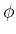be a predicate defined as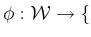TRUE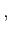FALSE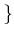, which returns TRUE for a point in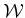that lies in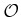, and FALSE otherwise. For a line given by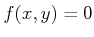, let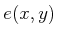denote a logical predicate that returns TRUE if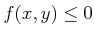, and FALSE otherwise.

A predicate that corresponds to a convex polygonal region is represented by a logical conjunction,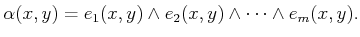(3.5)

The predicate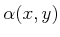returns TRUE if the point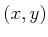lies in the convex polygonal region, and FALSE otherwise. An obstacle region that consists ofconvex polygons is represented by a logical disjunction of conjuncts,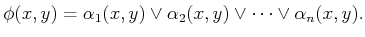(3.6)

Although more efficient methods exist,can check whether a pointlies inin time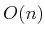, in whichis the number of primitives that appear in the representation of(each primitive is evaluated in constant time).

Note the convenient connection between a logical predicate representation and a set-theoretic representation. Using the logical predicate, the unions and intersections of the set-theoretic representation are replaced by logical ORs and ANDs. It is well known from Boolean algebra that any complicated logical sentence can be reduced to a logical disjunction of conjunctions (this is often called sum of products'' in computer engineering). This is equivalent to our previous statement thatcan always be represented as a union of intersections of primitives.

Steven M LaValle 2020-08-14